Himanshi Singh — March 5, 2021

## Introduction

We frequently use the term Probability but don’t realize how powerful this concept is. In simple terms, the probability is the likelihood or chance of something happening. And one of the fundamental concepts of probability is the Axioms of probability, which are essential for statistics and Exploratory Data Analysis.

Axioms mean a rule a principle that most people believe to be true. It is the premise on the basis of which we do further reasoning

In this article, I’m going to cover the Three Axioms of probability in detail.

Note: If you are more interested in learning concepts in an Audio-Visual format, We have this entire article explained in the video below. If not, you may continue reading.

## Axioms of Probability

There are three axioms of probability that make the foundation of probability theory-

#### Axiom 1: Probability of Event

The first one is that the probability of an event is always between 0 and 1. 1 indicates definite action of any of the outcome of an event and 0 indicates no outcome of the event is possible.

#### Axiom 2: Probability of Sample Space

For sample space, the probability of the entire sample space is 1.

#### Axiom 3: Mutually Exclusive Events

And the third one is- the probability of the event containing any possible outcome of two mutually disjoint is the summation of their individual probability.

Now let’s look at each one of them in detail!

## 1. Probability of Event

The first axiom of probability is that the probability of any event is between 0 and 1.As we know the formula of probability is that we divide the total number of outcomes in the event by the total number of outcomes in sample space.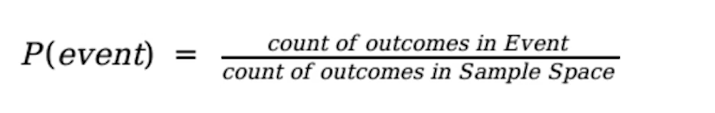And the event is a subset of sample space, so the event cannot have more outcome than the sample space. Clearly, this value is going to be between 0 and 1 since the denominator is always greater than the numerator.

## 2. Probability of Sample Space

The second axiom is that the probability for the entire sample space equals 1.Let’s take an example from the dataset. Suppose we need to find out the probability of churning for the female customers by their occupation type.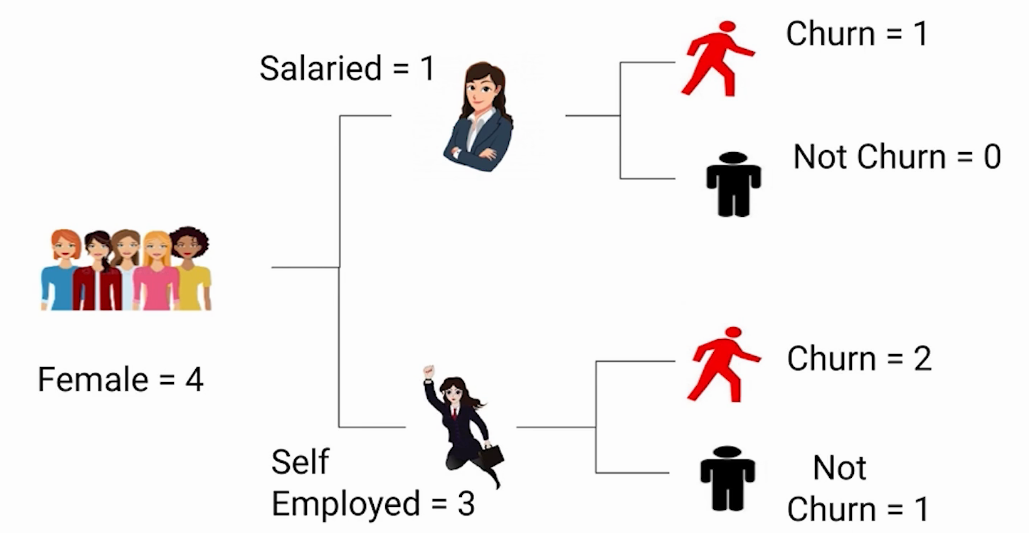In our data-set, we have 4 female customers, one of them is Salaried and three of them are self-employed. The salaried female is going to churn. Since we have only one salaried female who is going to churn, the number of salaried female customers who are not going to churn is 0. Amongst 3 self-employed female customers, two are going to churn and we can see that one self-employed female is not going to churn. This is the complete dataset: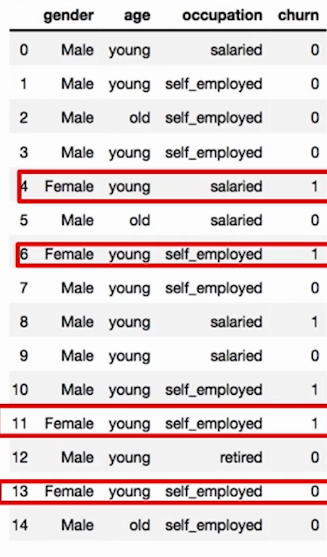So the probability of the churning status of female customer by profession, in the sample space of the problem we actually have:

Salaried Churn, Salaried Not churn, Self-employed Churn, Self-employed Not churn

And as we discussed their distribution earlier, in this sample space of female customer:

Salaried Churn = 1

Salaried Not churn = 0

Self-employed Churn = 2

Self-employed Not churn = 1

If you were to find out the probability that a person who is a female is salaried and is churning it will be equal to: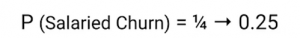Similarly, the probability of Salaried Not churn is: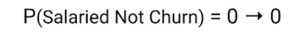Then we have Self-employed Churn: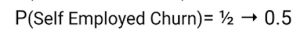And finally Self-employed Not Churn: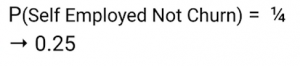And if we sum all of them up we get 1: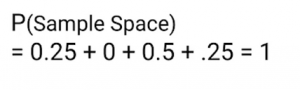So essentially saying that this is our entire sample space and the total probability that we get here is equals to 1. This brings us to axiom 3 which is related to mutually exclusive events.

## 3. Mutually Exclusive EventIf you remember the union formula you will recall that the intersection term is not here, which means there is nothing common between A and B. Let us understand these particular type of events which is called Mutually Exclusive Events.

These Mutually exclusive events mean that such events cannot occur together or in other words, they don’t have common values or we can say their intersection is zero/null. We can also represent such events as follows:This means that the intersection is zero or they do not have any common value. For example, if the

Event A:  is getting a number greater than 4 after rolling a die, the possible outcomes would be 5 and 6.Even B:  is getting a number less than 3 on rolling a die. Here the possible outcomes would be 1 and 2.Clearly, both these events cannot have any common outcome. An interesting thing to note here is that events A and B are not complemented of each other but yet they’re mutually exclusive.

## Mutually Exhaustive

One more important concept is Mutually Exhaustive Event which is often confused with mutually inclusive events. Mutually exhaustive events mean that such events together make up everything that can possibly happen in a random experiment. That means the union of these events makes the sample space: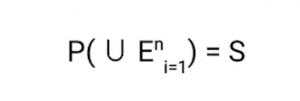Let’s understand this with an example-

Event A: Getting a number greater than 2 after a die, possible outcomes would be-Event B: Getting a number less than 4 after rolling a die. Here the possible outcomes would be:Clearly, both these events together make up all the outcomes that can possibly take place after rolling a die.

### How Mutually Exhaustive events differ from Mutually Exclusive events?

In the previous example getting a number 3 was common between both events. So these CANNOT be mutually exclusive but they definitely are mutually exhaustive. On the other hand, if we have another event:

Event C: Getting a number less than 3 after rolling a die, the possible outcomes would be:Now we can say that Event A and Event C are mutually exclusive since they have nothing in common.

## End Notes

In this article, we covered the axioms of probability and the difference between mutually exclusive and mutually exhaustive events.

If you are looking to kick start your Data Science Journey and want every topic under one roof, your search stops here. Check out Analytics Vidhya’s Certified AI & ML BlackBelt Plus Program

Let us know in the comments if you have any queries!